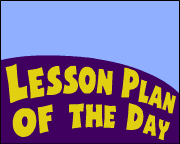## Search form# Fun Lesson: Math on the Move

Subjects

Mathematics
--Arithmetic
Physical Education
--Exercise/Movement

K-2
3-5
6-8
9-12

Brief Description Reinforce any math skill. Get kids moving with this math active-ity.

Objectives

• Students will actively engage their minds and bodies as they solve math problems.

Materials Needed

• a set of cards (card sets will vary according to the skill being taught/reinforced; see the Prepare the Lesson section below)

Lesson Plan

For some students -- especially boys -- being actively engaged and getting up-and-moving can be a difference maker when it comes to learning and reinforcing math skills. In this simple lesson idea, students will move around as they solve math problems for the missing number.

Prepare the Lesson
In advance of the lesson, you will need to create a series of cards with large numbers and math symbols on them. For the purpose of this example, we will assume that your class includes 25 students so you will create a series of 22 cards numbered 0 to 21 as well as three additional cards --

• one with an addition/plus sign (+) on it;
• one with a subtraction/minus (-) sign on it; and
• one with an equal sign (=) on it.

Each card should be at least 8-1/2 x 11 inches in size. Write all numbers and symbols large and bold so they can be seen from the back of the classroom.

Engaging Students in the Lesson
Call up students to arrange themselves in a variety of orders. Leave spaces where each box symbol [ ] appears below. Once you have arranged the students, call on the person holding the card with the missing number to come forward and fill in the box/space [ ] in the equation.

16  = 7
7 + 4 = 
20  = 18
7 +  = 13
13 3 = 

Continue to set up equations in this manner. Keep the activity moving quickly so that students are getting a little exercise of the body as well as the brain. After doing 10-15 equations, you might have students exchange cards so everyone has a different number or symbol before continuing the activity.

This lesson plan can be adapted in many ways. Prepare different sets of number cards to be used to teach or reinforce any math skill.

Example 1: The 12 Tables
Create a set of number cards to reinforce the 12 times tables. In this case, you would create 27 cards with all the numbers/symbols that would come into play when doing the 12 tables": 0, 1, 2, 3, 4, 5, 6, 7, 8, 9, 10, 11, 12, 24, 36, 48, 60, 72, 84, 96, 108, 120, 132, and 144 as well as the multiplication sign (X), division sign (/), and equal sign (=). If you have more cards than kids, some students might be given two cards. Call up students to arrange themselves in a variety of orders; then call for students holding the card with the missing number to come forward and fill in the box/space [ ] in the equation.

11 X  = 66
 / 11 = 9
3 X 11 = 
4 X  = 44
11 X 12 = 

Example 2: Adding a Column of Numbers
Prepare in advance a set of number cards for reinforcing column addition of three numbers. In this case you might create 25 cards that are numbered 0 to 21 as well as two plus signs (+) and one equal sign (=). Have students arrange themselves in a variety of ways and then have them fill in the missing number [ ].

6 + 5 + 4 = 
 + 6 + 8 = 21
16 + 2 + 3 = 
8 +  + 4 = 18
4 + 5 +  = 20
6 +  + 5 = 11

Example 3: Solving Equations for the Missing Number
Create a set of number cards to use in solving an equation for the missing number. In this case you might create 25 cards that are numbered 0 to 19 as well as two plus signs (+), two minus signs (-), and an equal sign (=). Have students arrange themselves in a variety of ways and then have them fill in the missing number [ ].

(6 + 5) - 4 = 
( + 5) + 7 = 16
4 ( - 5) = 3
19 ( 3) = 14
16 (2 + ) = 9
6 + (30 ) = 16

Assessment

Assess students based on their engagement and correct responses.

National Standards

MATHEMATICS: Number and Operations
NM-NUM.PK-2.1 Understand Numbers, Ways of Representing Numbers, Relationships Among Numbers, and Number Systems
NM-NUM.PK-2.2 Understand Meanings of Operations and How They Relate to One Another
NM-NUM.PK-2.3 Compute Fluently and Make Reasonable Estimates
NM-NUM.3-5.1 Understand Numbers, Ways of Representing Numbers, Relationships Among Numbers, and Number Systems
NM-NUM.3-5.2 Understand Meanings of Operations and How They Relate to One Another
NM-NUM.3-5.3 Compute Fluently and Make Reasonable Estimates
NM-NUM.6-8.1 Understand Numbers, Ways of Representing Numbers, Relationships Among Numbers, and Number Systems
NM-NUM.6-8.2 Understand Meanings of Operations and How They Relate to One Another
NM-NUM.6-8.3 Compute Fluently and Make Reasonable Estimates
NM-NUM.9-12.1 Understand Numbers, Ways of Representing Numbers, Relationships Among Numbers, and Number Systems
NM-NUM.9-12.2 Understand Meanings of Operations and How They Relate to One Another
NM-NUM.9-12.3 Compute Fluently and Make Reasonable Estimates

MATHEMATICS: Representation